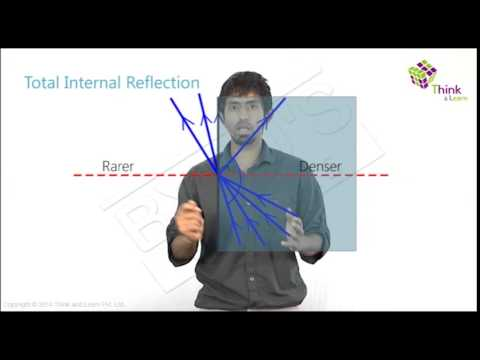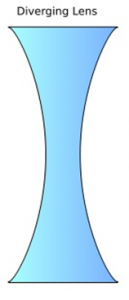# Refraction Of Light By Spherical LensesIn principle, spherical lenses, like spherical mirrors, are a part cut of from a bigger sphere. The refractive index of glass is higher than that of the surrounding air. Light rays passing from air to glass are obviously refracted. By using special types of spherical lenses, which are mainly convex lenses or concave lenses, the light rays passing from air to the lens in regard, are either diverged or converged. This application is put to many uses.

Lenses are used as part of the construction of telescopes and microscopes. They are also used in cameras and projectors. Your own eyes are an example of a spherical lens.Of course here, the image is inverted, but when the optical nerves transfer the impulse to the brain it processes the inverted image and you perceive the image in its correct orientation. FYI, if you’re seeing inverted images, nothing is wrong with your eyes… you’ll have to check your brain.

Getting back to our spherical lenses, the following terms may come your way when you are dealing with refraction in concave and convex lenses:

1. Centre of Curvature: The centre of the actual glass sphere, of which your lens forms a part
2. Principal Axis: When two spheres are part of your lens, it is the imaginary line joining the centres of curvatures of both spheres.
3. Principal Focus: It is point on the principal axis, where light rays parallel to principal axis meet in case of a convex lens (or appear to meet after extrapolation in case of a concave lens).
4. Optical Centre: It is a point within the lens where the diameter of the lens and the principal axis meet
5. Focal Length: The distance between the focus and the optical centre

## Convex Lens

A convex lens is also referred to as a converging lens since it “converges” light rays that are incident on it. The lens surface is convex in nature. The figure below shows a common biconvex lens used in labs.If a ray of light passes parallel to the principal axis of a convex lens, the refracted ray passes through the focus. If the incident ray passes through the optical centre, the refracted ray does not deviate at all. If the incident ray passes through the focus of the convex lens, the refracted ray then passes parallel to the principal axis.

## Concave Lens

A concave lens is also referred to as a diverging lens since it “diverges” light rays that are incident on it. The lens surface is concave in nature. The figure below shows a common biconcave lens used in labs.An incident light ray parallel to the principal axis of a concave lens passes through it and diverges. When extrapolated, it appears to pass through the focus. If the incident light ray passes through the optic centre, like in the convex lens, the refracted ray passes through without any deviation. If the incident light ray passes through the focus, it is refracted parallel to the principal axis.